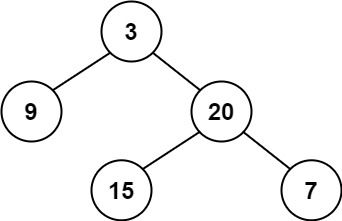top of page
Search

# Balanced Binary Tree

Given a binary tree, determine if it is height-balanced.

For this problem, a height-balanced binary tree is defined as:

a binary tree in which the left and right subtrees of every node differ in height by no more than 1.

Example 1:Example 1:

```Input: root = [3,9,20,null,null,15,7]
Output: true```

Example 2:```Input: root = [1,2,2,3,3,null,null,4,4]
Output: false

```

Example 3:

```Input: root = []
Output: true

```

Constraints:

• The number of nodes in the tree is in the range [0, 5000].

• -104 <= Node.val <= 104

class Solution { boolean isBalanced = true; public boolean isBalanced(TreeNode root) { helper(root); return isBalanced; } private int helper(TreeNode node) { if (node == null) return 0; int left = helper(node.left); int right = helper(node.right); if (Math.abs(left - right) > 1) { isBalanced = false; } return Math.max(left, right) + 1; } }